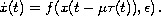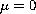Electron. J. Diff. Eqns., Vol. 2006(2006), No. 141, pp. 1-12.

### Periodic solutions for functional differential equations with periodic delay close to zero My Lhassan Hbid, Redouane Qesmi

Abstract:
This paper studies the existence of periodic solutions to the delay differential equationThe analysis is based on a perturbation method previously used for retarded differential equations with constant delay. By transforming the studied equation into a perturbed non-autonomous ordinary equation and using a bifurcation result and the Poincare procedure for this last equation, we prove the existence of a branch of periodic solutions, for the periodic delay equation, bifurcating from.

Submitted August 25, 2006. Published November 9, 2006.
Math Subject Classifications: 34K13.
Key Words: Differential equation; periodic delay; bifurcation; h-asymptotic stability; periodic solution.

Show me the PDF file (232K), TEX file, and other files for this article.

 My Lhassan Hbid Département de Mathématiques, Faculté des Sciences Semlalia Université Cadi Ayyad, B.P. S15, Marrakech, Morocco email: hassan.hbid@gmail.com Redouane Qesmi Département de Mathématiques, Faculté des Sciences Semlalia Université Cadi Ayyad, B.P. S15, Marrakech, Morocco email: qesmir@gmail.com